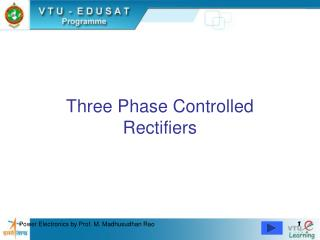Download PresentationThree Phase Controlled Rectifiers

# Three Phase Controlled Rectifiers

Download Presentation## Three Phase Controlled Rectifiers

- - - - - - - - - - - - - - - - - - - - - - - - - - - E N D - - - - - - - - - - - - - - - - - - - - - - - - - - -
##### Presentation Transcript

1. 3 Phase Controlled Rectifiers • Operate from 3 phase ac supply voltage. • They provide higher dc output voltage. • Higher dc output power. • Higher output voltage ripple frequency. • Filtering requirements are simplified for smoothing out load voltage and load current. 2

2. Extensively used in high power variable speed industrial dc drives. • Three single phase half-wave converters can be connected together to form a three phase half-wave converter. 3

3. 3-Phase Half Wave Converter(3-Pulse Converter) with RL LoadContinuous & ConstantLoad Current Operation 4

4. 5

5. 8

6. van vbn vcn van 9

7. Each thyristor conducts for 2/3 (1200) Constant Load Current io=Ia Ia Ia 10

8. To Derive an Expression for the Average Output Voltage of a 3-Phase Half Wave Converter with RL Loadfor Continuous Load Current 11

9. 12

10. 13

11. 14

12. 15

13. 16

14. 17

15. 18

16. 19

17. 3 Phase Half Wave Controlled Rectifier Output Voltage Waveforms For RL Load atDifferent Trigger Angles 20

18. =300 =600 21

19. =900 22

20. 3 Phase Half Wave Controlled Rectifier With R Load andRL Load with FWD 23

21. 24

22. 3 Phase Half Wave Controlled Rectifier Output Voltage Waveforms For R Load or RL Load with FWDatDifferent Trigger Angles 25

23. =00 =150 26

24. =300 =600 27

25. To Derive An Expression For The Average Or Dc Output Voltage Of A 3 Phase Half Wave Converter With Resistive Load Or RL Load With FWD 28

26. 29

27. 30

28. 31

29. 32

30. Three Phase Semiconverters • 3 Phase semiconverters are used in Industrial dc drive applications upto 120kW power output. • Single quadrant operation is possible. • Power factor decreases as the delay angle increases. • Power factor is better than that of 3 phase half wave converter. 33

31. 3 Phase Half Controlled Bridge Converter (Semi Converter)with Highly Inductive Load & Continuous Ripple free Load Current 34

32. 35

33. 37

34. 38

35. 39

36. 40

37. 41

38. 42

39. 43

40. 45

41. 46

42. 47

43. To derive an Expression for the Average Output Voltage of 3 Phase Semiconverter for  >  / 3 and Discontinuous Output Voltage 48

44. 49

45. 50